# The ZF axioms

Zermelo and Fraenkel expressed their axioms in the formal language of set theory, but we can translate them into ordinary language.

Axiom 1: Two sets are equal if and only if they have the same elements.

Axiom 2: There is a set with no elements called the empty set.

Axiom 3: For any sets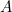and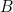there is a set whose only elements areand. (Note that taking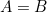, this gives you the possibility of forming the singleton set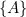.)

Axiom 4: For any setthere's a set whose elements are the elements of the elements of A. (If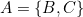then this set is what's called the union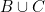ofand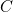, whose elements are all the elements oftogether with the elements of. For example if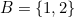and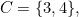then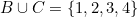.)

Axiom 5: For any setthere is a set, called the power set of, whose elements are the subsets of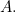(A subset ofis a set whose elements are all contained in. Power sets are an important ingredient of Cantor's hierarchy of infinities.)

Axiom 6: For any function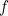defined on a set, the values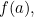where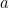is an element of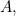also form a set.

Axiom 7: Every non-empty sethas a member whose elements are all different from the elements of. (This axiom insures that if you take an elementofand then an elementof, and so on, this process stops after finitely many steps.)

Axiom 8: There is an infinite set such that ifis in this set, the set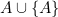is also in the set.

Back to main article